# 红黑树的插入详解及Javascript实现方法示例

更新时间：2018年03月26日 11:09:57   作者：nbb3210我要评论

1. 每个结点或是黑色或是红色。
2. 根结点是黑色的。
3. 每个叶结点(NIL)是黑色的。
4. 如果一个结点是红色的，则它的两个子结点都是黑色的。
5. 对每个结点，从该结点到其所有后代叶结点的简单路径上，均包含相同数目的黑色结点。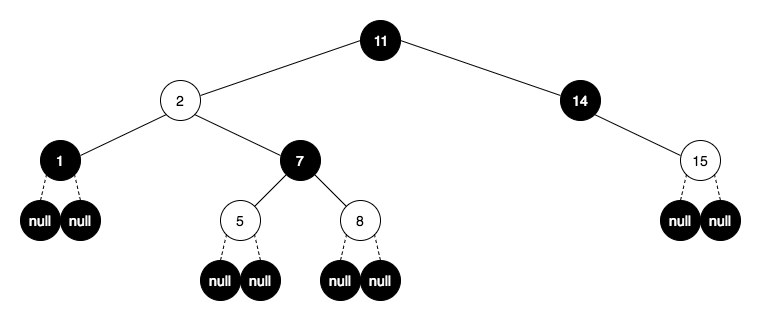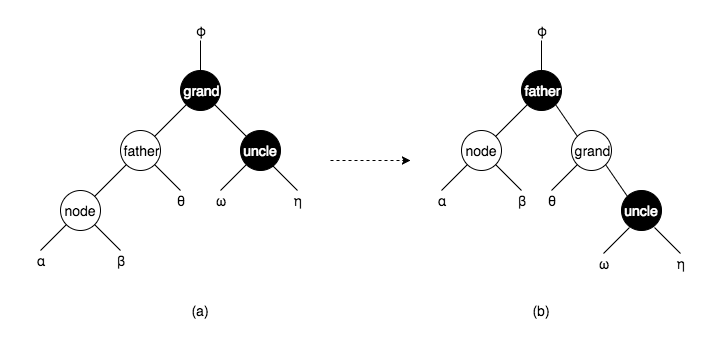node 为红，father 为红，grandfather 和 uncle 为黑的具体情形一

node 为红，father 为红，grandfather 和 uncle 为黑的具体情形二

node 为 father 的右子结点， father 为 grand 的右子结点，如下图(e)，就是具体情形一的翻转。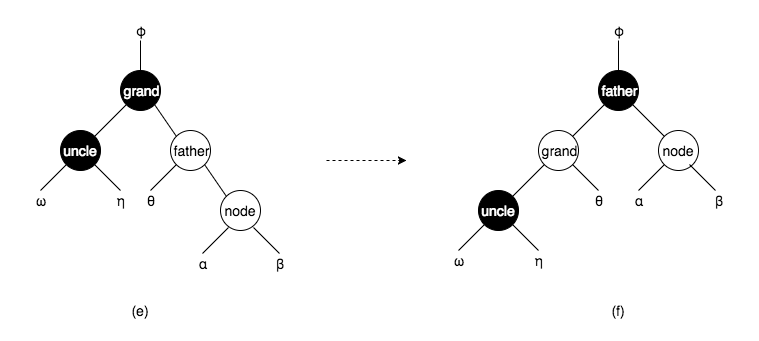node 为红，father 为红，grandfather 和 uncle 为黑的具体情形三

node 为 father 的右子结点， father 为 grand 的左子结点，如下图(m)。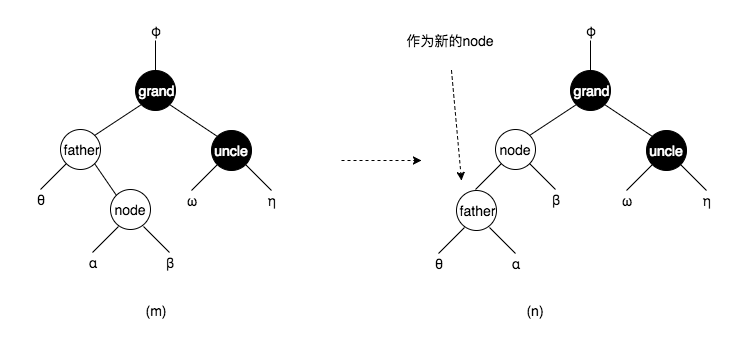node 为红，father 为红，grandfather 和 uncle 为黑的具体情形四

node 为 father 的右子结点， father 为 grand 的左子结点，如下图(i)，就是具体情形三的翻转。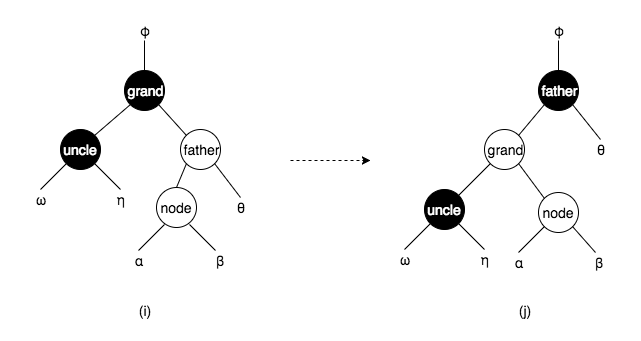node ，father 和 uncle 为红，grandfather 为黑。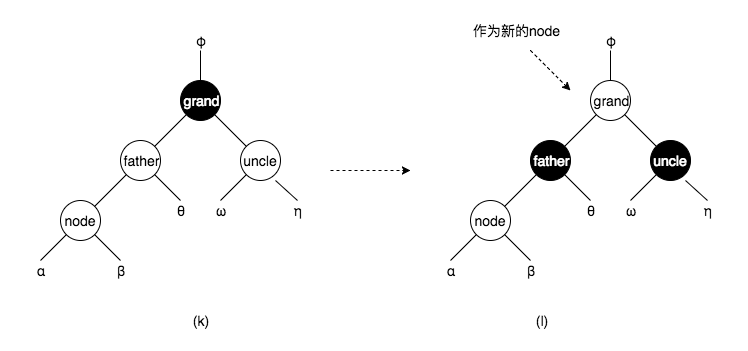node的情形 操作

```// 结点
function Node(value) {
this.value = value
this.color = 'red' // 结点的颜色默认为红色
this.parent = null
this.left = null
this.right = null
}

function RedBlackTree() {
this.root = null
}

RedBlackTree.prototype.insert = function (node) {
// 以二叉搜索树的方式插入结点
// 如果根结点不存在，则结点作为根结点
// 如果结点的值小于node，且结点的右子结点不存在，跳出循环
// 如果结点的值大于等于node，且结点的左子结点不存在，跳出循环
if (!this.root) {
this.root = node
} else {
let current = this.root
while (current[node.value <= current.value ? 'left' : 'right']) {
current = current[node.value <= current.value ? 'left' : 'right']
}
current[node.value <= current.value ? 'left' : 'right'] = node
node.parent = current
}
// 判断情形
this._fixTree(node)
return this
}

RedBlackTree.prototype._fixTree = function (node) {
// 当node.parent不存在时，即为情形1，跳出循环
// 当node.parent.color === 'black'时，即为情形2，跳出循环
while (node.parent && node.parent.color !== 'black') {
// 情形3
let father = node.parent
let grand = father.parent
let uncle = grand[grand.left === father ? 'right' : 'left']
if (!uncle || uncle.color === 'black') {
// 叶结点也是黑色的
// 情形3.1
let directionFromFatherToNode = father.left === node ? 'left' : 'right'
let directionFromGrandToFather = grand.left === father ? 'left' : 'right'
if (directionFromFatherToNode === directionFromGrandToFather) {
// 具体情形一或二
// 旋转
this._rotate(father)
// 变色
father.color = 'black'
grand.color = 'red'
} else {
// 具体情形三或四
// 旋转
this._rotate(node)
this._rotate(node)
// 变色
node.color = 'black'
grand.color = 'red'
}
break // 完成插入，跳出循环
} else {
// 情形3.2
// 变色
grand.color = 'red'
father.color = 'black'
uncle.color = 'black'
// 将grand设为新的node
node = grand
}
}

if (!node.parent) {
// 如果是情形1
node.color = 'black'
this.root = node
}
}

RedBlackTree.prototype._rotate = function (node) {
// 旋转 node 和 node.parent
let y = node.parent
if (y.right === node) {
if (y.parent) {
y.parent[y.parent.left === y ? 'left' : 'right'] = node
}
node.parent = y.parent
if (node.left) {
node.left.parent = y
}
y.right = node.left
node.left = y
y.parent = node
} else {
if (y.parent) {
y.parent[y.parent.left === y ? 'left' : 'right'] = node
}
node.parent = y.parent
if (node.right) {
node.right.parent = y
}
y.left = node.right
node.right = y
y.parent = node
}
}

let arr = [11, 2, 14, 1, 7, 15, 5, 8, 4, 16]
let tree = new RedBlackTree()
arr.forEach(i => tree.insert(new Node(i)))
debugger```